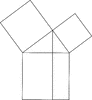# Euclid's Pythagorean Theorem ProofIllustration used to prove the Pythagorean Theorem, according to Euclid. A perpendicular is drawn from the top vertex of the right triangle extended through the bottom square, forming 2 rectangles. Each rectangle has the same area as one of the two legs. This proves that the sum of the squares of the legs is equal to the square of the hypotenuse (Pythagorean Theorem).

### Galleries

Geometric Theorems and Proofs

### Source

Florida Center for Instructional Technology Clipart ETC (Tampa: University of South Florida, 2007)

EPS (vector)

343.2 KiB

TIFF (full resolution)

2770×3000, 142.4 KiB

Large GIF

945×1024, 19.1 KiB

Medium GIF

590×640, 11.6 KiB

Small GIF

295×320, 5.5 KiB